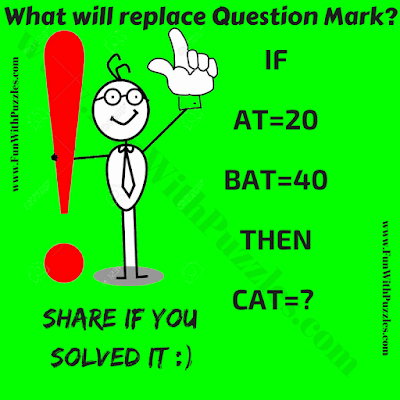## Monday, July 16, 2018

This Maths Puzzle Question for Kids is very easy one. In the given Puzzle Picture, there are few equations. Your challenge is find the logic in these equations and then find the value of CAT to get the number which will replace the question mark. Lets see how much time you will take to solve this Maths Puzzle Question?Can you find the value of CAT?

Answer of this "Maths Puzzle Question for Kids", can be viewed by clicking on button. Please do give your best try before looking at the answer.

1.1.